# Understanding Big-O Notation

It’s important when you’re writing applications especially, those that are going to be processing a large amount of data that you understand how your application is going to scale.

Big-O notation gives us a really simple way to understand how many operations your application needs to perform, depending on how much data it needs to process.

There are a few different, Big-O notations that you’re likely to come across, but the main ones are:

• Constant - O(1)
• Logarithmic - O(log n)
• Linear - O(n)
• Exponential - O(2^n)
• Factorial - O(n!)

If we plot these on a graph, we can quite clearly see how the number of operations that need to be performed increases with the number of elements that are being processed.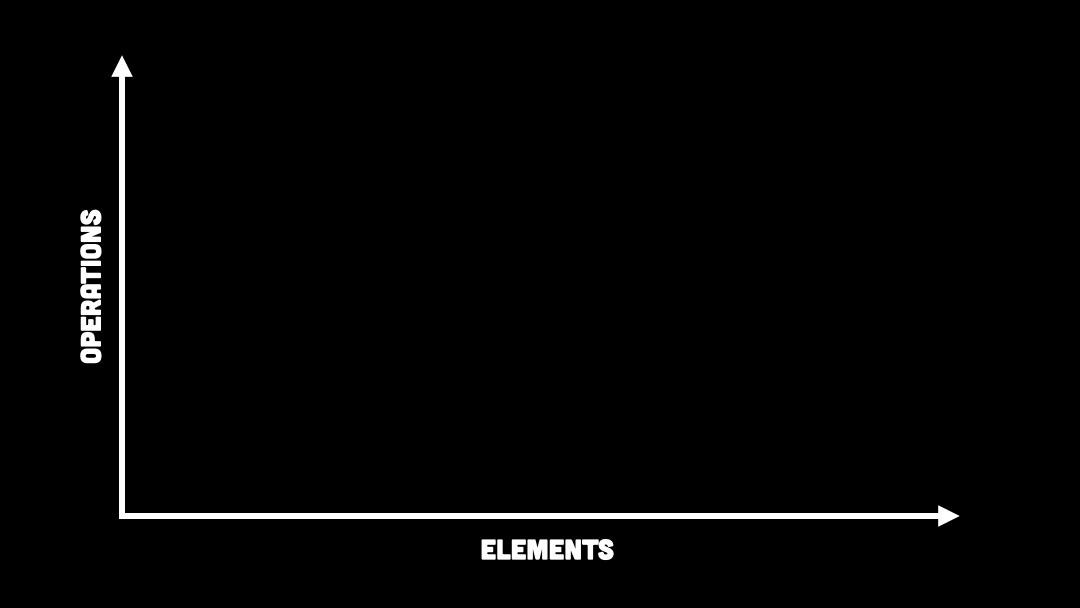You can see with quadratic, exponential, and factorial that the number of operations rapidly increases the more elements you need to process.

With the number of operations rapidly increasing, the time it’s going to take to run your application is going to increase rapidly as well.

## Constant - O(1)

Constant is anything that isn’t going to change depending on the number of elements that you have to process.

If there’s a particular part of your code that runs the same number of times, no matter how big your dataset is, then that is considered constant.

Even a loop can be considered constant if it’s running the same number of times, no matter how big the dataset is.

``````Console.WriteLine("Constant Example");
Console.WriteLine("Another Line");
Console.WriteLine("Another Line");
Console.WriteLine("Another Line");

for (int i = 0; i < 10; i++)
{
Console.WriteLine(i);
}``````

Technically every single line of code should go towards the time complexity, but when we’re calculating it, we don’t really care about constants.

Even if you have four lines of code that always run once we don’t calculate this as O(4), we just consider that to be O(1). This is because we’re looking for the big-picture calculation and a few additional lines of code with constant complexity aren’t going to affect the overall calculation that much.

We’re trying to get a sense overall of how your application is going to perform the larger the dataset it has to process.

##### 🚀 Are you looking to level up your engineering career?

You might like my free weekly newsletter, The Curious Engineer, where I give career advice and tackle complex engineering topics.

## Logarithmic - O(log n)

The logarithmic time complexity is the most efficient one we’re going to look at.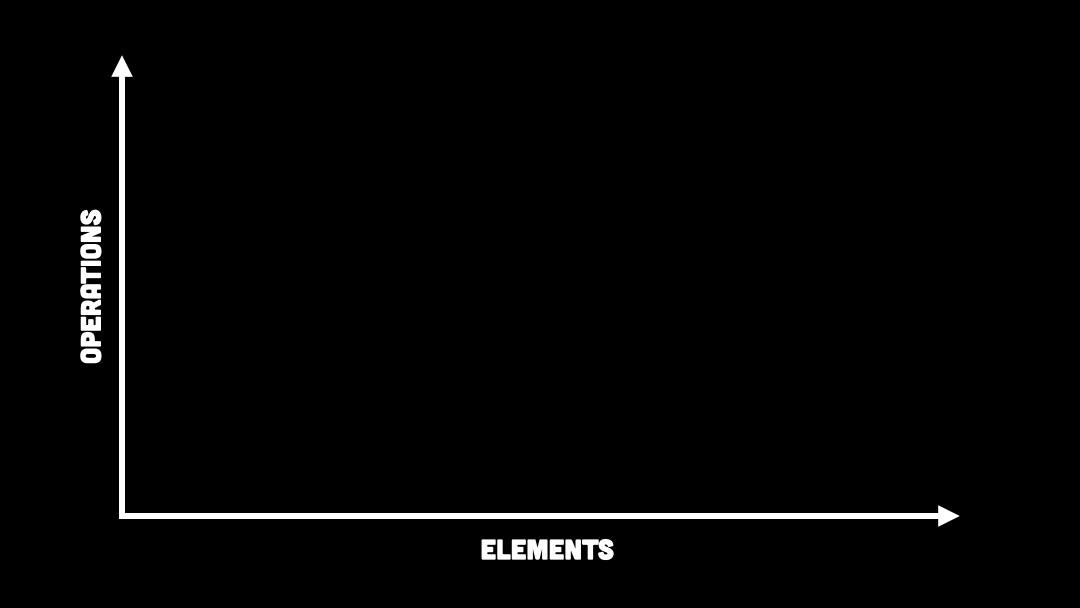You can see no matter how many items that we are processing, the time it takes and the number of operations that it needs to process that data doesn’t increase at the same rate.

The typical example you see for O(log n) is the binary search algorithm.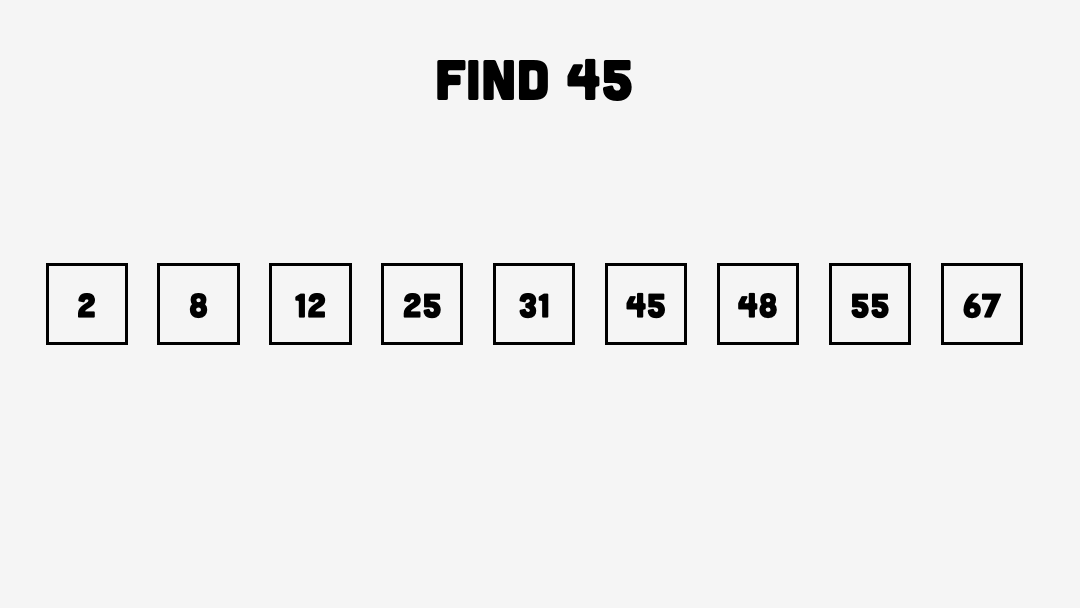To perform a binary search algorithm, we take a list of items and put them in size order.

To find the item that we’re looking for, we pick the middle number and see if it’s bigger or smaller than the number that we are looking for.

If the number we’re looking for is bigger then we can discard the smaller half of our dataset and then look at the larger half.

We keep looking at the middle number and keep discarding the other half until we get to the number that we are looking for.

You can see with each iteration, we are halving the dataset. So even with very large datasets, you can get through them very quickly when you’re halving the set each time.

## Linear - O(n)

As the name suggests, the number of operations that your application needs to perform is going to scale linearly with the size of the dataset.

If we take our search example, instead of using a binary search algorithm, we could just loop through the whole dataset.

Worst case scenario, if the number you’re looking for can’t be found, then you’ve still got to loop through every single item in the dataset and therefore it’s going to take N operations before your application completes.

Of course, you could get lucky and the number you are looking for is actually the first or second number in your array. But when calculating big O notation, we’re always looking for the worst-case scenario.

It’s important when we’re calculating big O notation that we always discard static multiples. Say, for example, you have two loops, each of them processing from 0 to N and each one running one after another.

``````var n = 10;

for (int i = 0; i < n; i++)
{
Console.WriteLine(i);
}

for (int i = 0; i < n; i++)
{
Console.WriteLine(i);
}``````

Technically, this should be calculated as 2N, because we’re going through two loops, each processing N number of elements.

But when we’re calculating Big-O notation, we always drop those multiples. We just want to get an overview of how your code is performing.

However, if you are trying to optimise your code, you should definitely look at trying to remove those additional loops.

This is the case when we’ve got two loops that are running one after the other. If we have one of those loops nested inside the other one, then that definitely does count towards the time complexity.

Whenever you have a loop inside a loop, this is likely to be quadratic time complexity.

Now to see N^2 in action, we can have a look at this code example.

``````var n = 10;

for (int i = 1; i <= n; i++)
{
for (int j = 1; j <= n; j++)
{
Console.Write("0 ");
}

Console.WriteLine();
}``````

Each loop is going from 1 to N with the inner loop printing out 0 and the outer loop, just printing out a new line.

Now the good thing about this example is we can actually see this is quite clearly N^2 when we print out the output.It’s not actually that common to have the inner loop looping the same number of times as the outer loop. What’s more common is that the inner loop might loop to the index you’re currently on.

``````var n = 10;

for (int i = 1; i <= n; i++)
{
for (int j = 1; j <= i; j++)
{
Console.Write("0 ");
}

Console.WriteLine();
}``````

In this example, we have got the outer loop `i` going from 1 to 10, but the inner loop `j` is going from 1 to `i`.

If we print this out, we can see that the number of times the inner loop is running is going to be increasing each time.We can see from the output that it is quite clear that this is going to be N^2/2 because it’s half the size of what we had before.

However, as we always discard constant multiples, and in this case, the multiple is half, this still ends up being O(n^2) when we calculate the time complexity for it.

## Exponential - O(2^n)

You can see when we look at this on a graph, if your application is scaling exponentially, that’s going to be pretty bad for your application.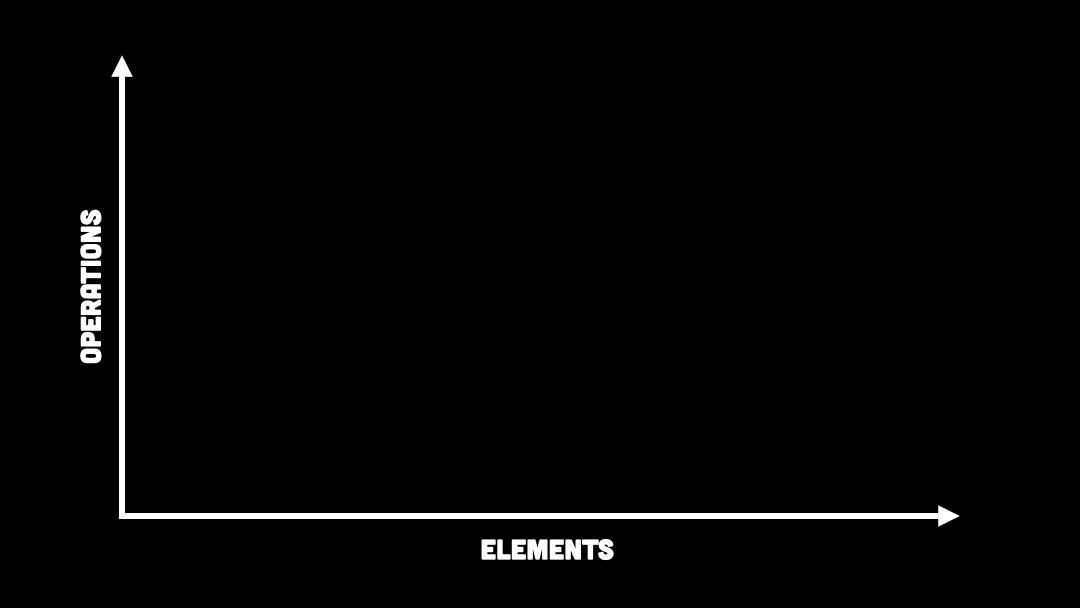It’s going to have to run through lots of operations as your dataset increases and the time it takes for your application to run is going to increase as well.

The most common example you will see when looking at exponential time complexity is calculating the Fibonacci sequence.

Here we have a function to calculate the numbers in the Fibonacci sequence.

``````int fib(int num)
{
if (num <= 1) return num;
return fib(num - 2) + fib(num - 1);
}``````

Say we want to calculate the 6th number in the Fibonacci sequence.

You can see here that if we put in 6, the code is going to be making two calls to itself. The easiest way to picture this is by putting it into a binary tree.This is treated as 2^n because for every single level we go down we’re doubling the number of calls that we need to make.

Now, if we have a look at our code, we can see we have an additional return statement.

If the number is one or less, then we’re going to return the input number. This actually puts a cap on the number of times the function is going to call itself.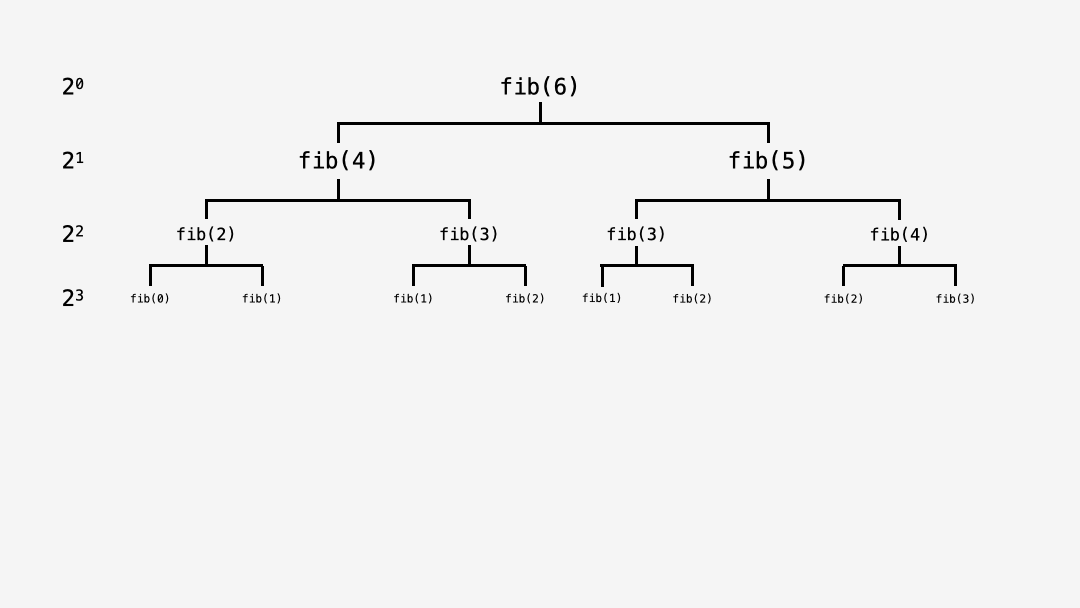We can see when we go down to the next level, the number we’re putting in is one or zero, and then the function is not going to make any further calls to itself.

We can see that it’s never going to be 2^n as we aren’t always doubling the calls each time.

Those familiar with the Fibonacci sequence will know that it’s closely related to the golden ratio, which is 1.618.The Fibonacci sequence is not therefore 2^n, but 1.618^n.

## Calculating time complexity in your code

When you’re trying to calculate the Big-O notation for your own code, you don’t want to be going through every single function and trying to work out how it scales.

Luckily, for the most common functions, such as search algorithms, it is already common knowledge what the Big-O notation is for these.

For example:

• Binary Search - O(log n)
• Quick Sort - O(n log n)
• Adding to a Stack - O(1)
• Searching Linked List - O(n)
• Comparing Strings - O(n)
• Calculating Fibonacci Sequence - O(2^n)
🙏 Was this helpful? If you want to say thanks, I love coffee ☕️ , any support is appreciated.